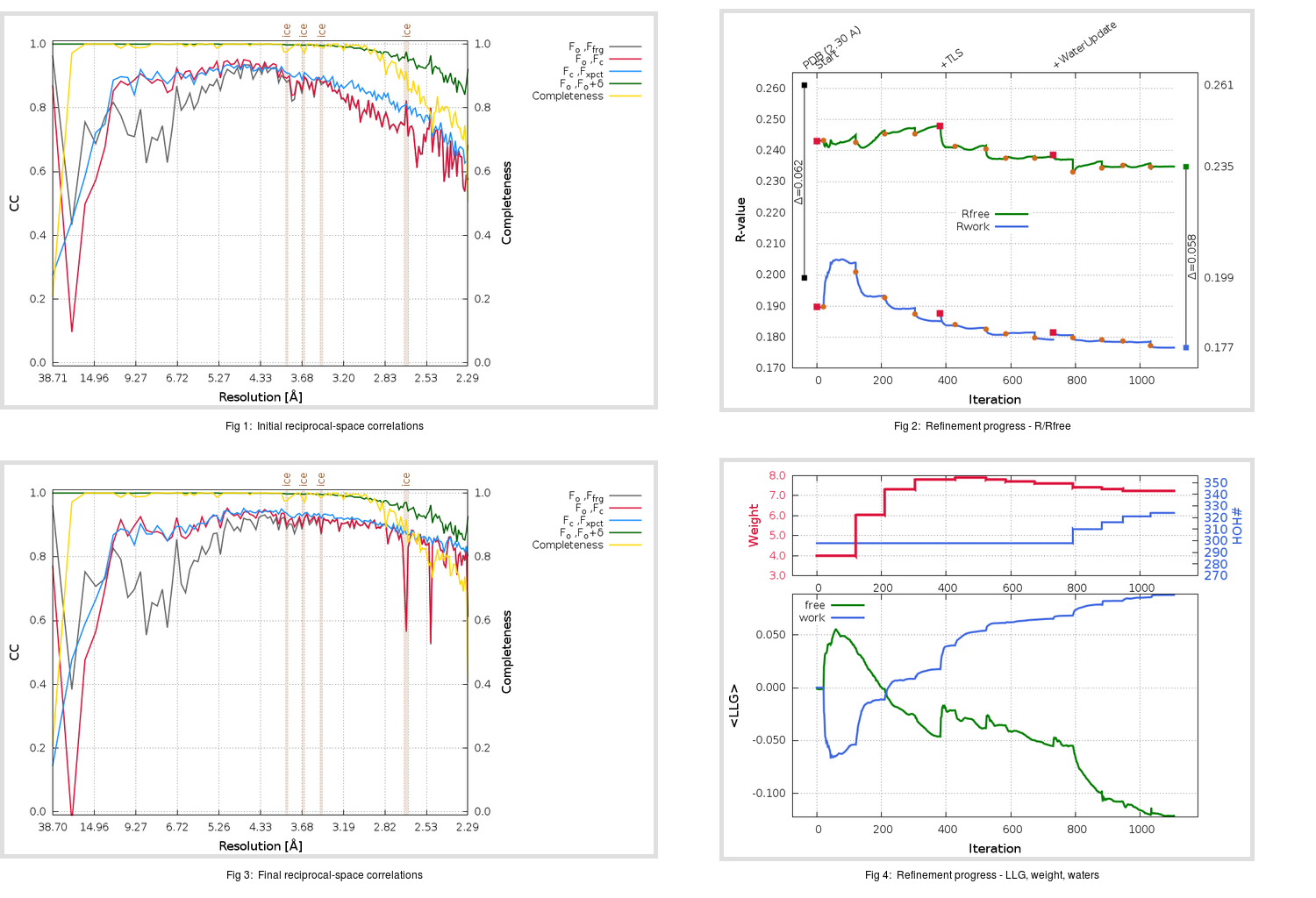Content:

```    Diffraction limits & principal axes of ellipsoid fitted to diffraction cut-off surface:
2.173         0.9662   0.0000  -0.2579       0.894 a* - 0.448 c*
2.228         0.0000   1.0000   0.0000       b*
2.629         0.2579   0.0000   0.9662       0.221 a* + 0.975 c*
```

## Deposited

` `
 Date deposited Date data collection Resolution R, Rfree 20060124 20051014 2.30 0.1990 0.2610

Molprobity (CCP4 7.0 version) summary:

```Ramachandran outliers =   0.81 %
favored =  92.07 %
Rotamer outliers      =   4.51 %
C-beta deviations     =     0
Clashscore            =  14.88
RMS(bonds)            =   0.0062
RMS(angles)           =   1.34
MolProbity score      =   2.66
Resolution            =   2.30
R-work                =   0.1990
R-free                =   0.2610
```

```Number of waters      =   298

<B> (all atoms) =   49.69 ( sd =   19.57 ) for       5057 non-hydrogen atoms
<B>   (protein) =   49.60 ( sd =   19.89 ) for       4740 non-hydrogen atoms
<B>     (water) =   48.73 ( sd =   10.70 ) for        298 non-hydrogen atoms
<B>    (others) =   86.79 ( sd =   10.45 ) for         19 non-hydrogen atoms

B min/max       (all non-hydrogen atoms) =    4.92 /  100.64
B min/max   (protein non-hydrogen atoms) =    4.92 /  100.64
B min/max     (water non-hydrogen atoms) =   25.63 /   79.41
B min/max     (other non-hydrogen atoms) =   67.54 /   98.97
```

## BUSTER (re-)refinement

` `

Molprobity (CCP4 7.0 version) summary:

```Ramachandran outliers =   0.32 %
favored =  95.63 %
Rotamer outliers      =   7.14 %
C-beta deviations     =     4
Clashscore            =   4.71
RMS(bonds)            =   0.0112
RMS(angles)           =   1.61
MolProbity score      =   2.19
Resolution            =   2.29
R-work                =   0.1767
R-free                =   0.2349
```

```Number of waters      =   324

<B> (all atoms) =   51.41 ( sd =   16.88 ) for       5083 non-hydrogen atoms
<B>   (protein) =   51.24 ( sd =   17.15 ) for       4740 non-hydrogen atoms
<B>     (water) =   52.51 ( sd =   11.53 ) for        324 non-hydrogen atoms
<B>    (others) =   73.82 ( sd =    7.42 ) for         19 non-hydrogen atoms

B min/max       (all non-hydrogen atoms) =   24.07 /  115.87
B min/max   (protein non-hydrogen atoms) =   24.07 /  115.87
B min/max     (water non-hydrogen atoms) =   28.03 /   94.42
B min/max     (other non-hydrogen atoms) =   61.15 /   79.35
```

Refinement progression:Results:

` `
 File Remark 2DD8_aB_refine.01_03_refine.pdb.gz exact refinement commands are in header 2DD8_aB_refine.01_03_refine.mtz.gz including original deposited data and several re-refinement map coefficients 2DD8_aB_refine.01_03_BUSTER_model.cif.gz including any non-standard compound restraints 2DD8_aB_refine.01_03_BUSTER_refln.cif.gz